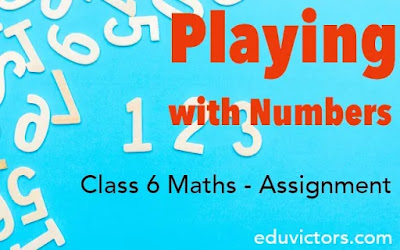# Class 6 - Maths - Playing With Numbers (Assignment)

Fill in the blanks with suitable word(s).

1. Numbers having more than two factors are called _____________ numbers.

2. A _______ of a number is an exact divisor of that number.

3. ______ is a factor of every number.

4. ______ is the smallest prime number.

5. The smallest odd prime number is ____________.

6. The factors of a given number are always ______ than or equal to the number.

7. ___________ is neither a prime nor composite number.

8. Every multiple of a number is ___________ than or equal to the number.

9. If the sum of all the factors of any number is equal to the double of that number then that number is called a _________ Number.

10. If the last digit of a number is even, then the number is divisble by _____.

11. If a number has _____ in ones place, then it is divisible by 10.

12. If the sum of the digits in a number is a _____ of 3, then the number is divisible by 3.

13. If 1 is the only common factor between two numbers then they are said to be __________ Numbers.

14. HCF of 24 and 36 is ______

15. LCM of 24 and 36 is ______

HOTS Question: A book has 380 pages. How many digits are used to print the page numbers of the book?

Page 1 to 9 =  9 digits

Page 10 to 99 =  180 digits

Page 100 to 300 =  300 - 100 + 1 = 201 pages

=  201 × 3 = 603 digits

Total digits = 9 + 180 + 603 = 792 digits (Answer)

1. composite

2. factor

3. 1 (One)

4. 2 (Two)

5. 3 (three)

6. less

7. One (1)

8. more

9. Perfect

10. 2

11. 0

12. multiple

13. Co-prime

14. 12

15. 72